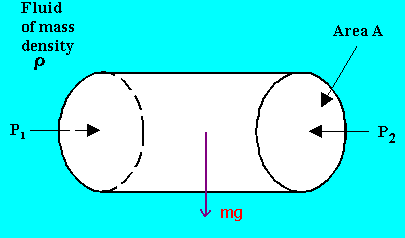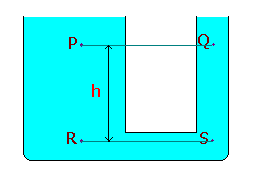### Variation of pressure with elevation

Home -> Lecture Notes -> Fluid Mechanics -> Unit-IConsider a hypothetical differential cylindrical element of fluid of cross sectional area A and height (z2 - z1).

Upward force due to pressure P1 on the element = P1A

Downward force due to pressure P2 on the element = P2A

Force due to weight of the element = mg = rA(z2 - z1)g

Equating the upward and downward forces,

P1A = P2A + rA(z2 - z1)g

P2 - P1 = - rg(z2 - z1)

Thus in any fluid under gravitational acceleration, pressure decreases, with increasing height z in the upward direction.

Equality of pressure at the same level in a static fluid:Equating the horizontal forces, P1A = P2A (i.e. some of the horizontal forces must be zero)

Equality of pressure at the same level in a continuous body of fluid:Pressures at the same level will be equal in a continuous body of fluid, even though there is no direct horizontal path between P and Q provided that P and Q are in the same continuous body of fluid.

We know that, PR = PS

PR = PP + rgh à 1

PS = PQ + rgh à 2

From equn.1 and 2, PP = PQ

General equation for the variation of pressure due to gravity from point to point in a static fluid:Resolving the forces along the axis PQ,

pA - (p + dp)A - rgAds cos(q) = 0

dp = - rgds cos(q)

or in differential form,

dp/ds = - rgcos(q)

In the vertical z direction, q = 0.

Therefore,

dp/dz = -rg

This equation predicts a pressure decrease in the vertically upwards direction at a rate proportional to the local density.